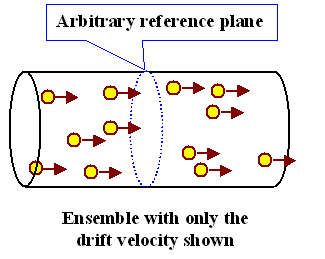# Mechanism of current flow in a metallic conductor

We know that a conductor is a material which comprises movable or non-static electric charges. We shall discus the mechanism of metallic conductors. In case of conductors which are metallic, these charged particles are electrons. Besides these, positive charges can also be in the form of ions like in the electrolyte of a battery. Insulators are the materials which have less mobile charges and oppose the flow of current. Thus, all the conductors contain electric charges but these charges move only when some potential difference is created across two diverse points on the conductor. This charge flow is measured in amperes and is termed as electric current.

In a majority of materials, as confirmed by Ohm’s Law, direct current is proportional to the voltage provided there is no change in the shape and state of material and the temperature also remains constant. Copper is a very good conductor and hence is normally used for electrical wiring. Besides these, there are numerous non-metallic conductors like graphite, solutions of salts and plasmas.

## Definition and Mechanism of Electric Current

In simple words, electric current is a movement or flow of electrically charged particles and its unit of measurement is amperes. When an electric current flows in a conductor, it flows as a drift of free electrons in the metal. Electricity flows easily through a conductor because the electrons are free to move around in the object. Whenever there is a movement of electrons through a conductor, an electric current is created. Current can also be divided into two types- DC and AC.

Dc or Direct Current- As the name suggests, the current that always flows in one direction and does not move back and forth is called as the direct current. The most common example of DC is battery.

AC or Alternating Current- The current that flows back and forth and not in one direction is termed as the alternating current.

Before we actually discuss the mechanism of current flow in a conductor we explain the concept of drift velocity as it will be required in its description.

Drift Velocity: The average velocity achieved by some particle like the electron due to the existence of electric field is termed as drift velocity. The particles are actually assumed to be moving along some plane and hence the velocity may also be termed as axial drift velocity.## Mechanism of Current Flow in a Conductor

We shall now discuss the advanced mechanism of electric current. Flow of current in metals is due to preferential flow of free electrons. In the absence of any externally applied emf (by means of a battery), the free electrons move randomly through the metal from one point to another giving zero net current.

When connected to a battery, the free electrons get accelerated due to the electric field (set up by the battery) and they gain velocity and energy. However, the passage is not smooth and the electrons collide with the lattice ion in which the ultimate gainer (of energy) is the ion. As we know the temperature of a body is related with the energy of vibrations of these ions, these collisions result in increase in temperature of the metal. The loss of energy of electrons in collision and their acceleration by the electric field, finally, results in drifting of electrons in a particular direction. (Although the actual motion of electrons is erratic, the overall effect is of drifting of electrons).

The motion of the conducting electrons in an electric field is thus a combination of the motion due to random collisions. When we consider all the free electrons, their random motion average to zero and make no contribution to the drift speed. Thus, the drift speed is only due to the effect of the electric field on the electrons.

Watch this video for more on flow of electric current

askIITians offers comprehensive study material which contains all the important topics of IIT JEE Physics. All the areas like the flow of current through a metallic conductor or the mechanism of current flow have also been covered in the content. It is important for the JEE aspirants to have a strong grip on the topic in order to remain competitive in the JEE.

## Related Resources

To read more, Buy study materials of Current Electricity comprising study notes, revision notes, video lectures, previous year solved questions etc. Also browse for more study materials on Physics here.

### Course Features

• Video Lectures
• Revision Notes
• Previous Year Papers
• Mind Map
• Study Planner
• NCERT Solutions
• Discussion Forum
• Test paper with Video Solution